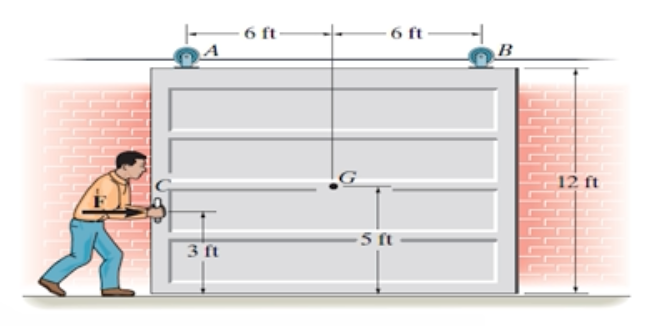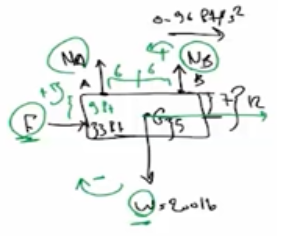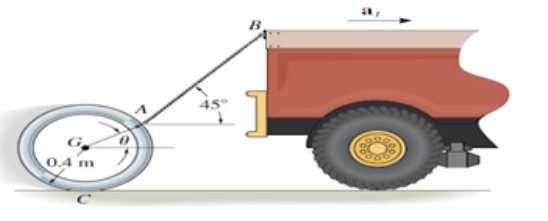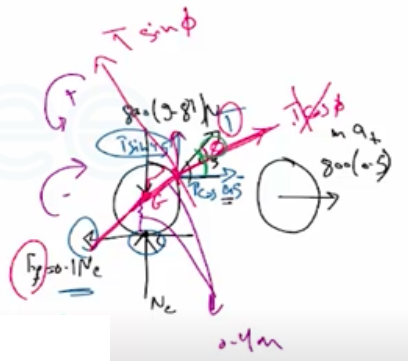Need Help?

Subscribe to Dynamic

###### \${selected_topic_name}
• Notes

$\begin{array}{l}{17-25 . \text { The door has a weight of } 2001 \mathrm{b} \text { and a center of }} \\ {\text { gravity at } G . \text { Determine the constant force } F \text { that must be }} \\ {\text { applied to the door to push it open } 12 \mathrm{ft} \text { to the right in } 5 \mathrm{s} \text { , }} \\ {\text { starting from rest. Also, find the vertical reactions at the }} \\ {\text { rollers } A \text { and } B \text { . }}\end{array}$$w d=200 l b$

$F=? ?$

$5=12 \mathrm{ft}$

$t=5s$

$S_{0}=0$

$V_{0}=0$

$\stackrel{+}{\rightarrow}\ s=s_0+v_{0} t+\frac{1}{2} a_{G} t^{2}$

$12=0+0+\frac{1}{2} a_{G}(5)^{2}$

$a_{G}=0.96 ft/s^{2}$$\stackrel{+}{\rightarrow}\ \Sigma F_{x}=m\left(a_{G}\right)_{x} \Rightarrow F=\frac{20 0}{32.2}(0.96)=5.96 \mathrm{lb}$

$\Sigma M_{A}=\Sigma\left(M_{k}\right)_{A} \Rightarrow N_{B}(12)-200(6)+5.96(9)$

$m\left(a_{G}\right)_{x}d_{z}=\frac{200}{32.2}(0.96) 7\$

$N B=9 9 lb$

$+\uparrow \Sigma F_ y=m(a_G)_y$

$N_{A}+99-200=0$

$N_ A=101l b$

$\begin{array}{l}{\text { 17-51. The pipe has a mass of } 800 \mathrm{kg} \text { and is being towed }} \\ {\text { behind the truck. If the acceleration of the truch is }} \\ {a_{t}=0.5 \mathrm{m} / \mathrm{s}^{2} \text { . determine the angle } \theta \text { and the tension in the }} \\ {\text { cable. The coefficient of kinetic friction between the pipe }} \\ {\text { and the ground is } \mu_{k}=\text { o.1. }}\end{array}$$m _p=800 k g$

$a_{t}=0.5 \mathrm{m} / \mathrm{s}^{2}$

$\theta=? ?$

$T=??$

$\mu_{k}=\mathbf{0} . \mathbf{1}$$\stackrel{+}{\rightarrow}\ \Sigma F_{x}=m a_{x}$

${-0.1 N_{c}+T{\cos } 45=800(0.5)\rightarrow(1)}\$

$+\uparrow \Sigma F_{y}=m a_ y$

$N_ c-800(9.81)+T \sin 45^{\circ}=0 \rightarrow (2)$

$N _c=6770.9 \mathrm{N} \ , T=1523.24 \mathrm{N}=1.52 \mathrm{kN}$

$\Sigma M_{G}=0$

$-0.1 N_{c}(0.4)+T \sin \phi(0.4)=0$

$\sin \phi=\frac{0.1(6770.9)}{1523. 24} \Rightarrow \phi=26. 39^{\circ}$

$\theta=45-26.39^{\circ}=18.6^{\circ}$